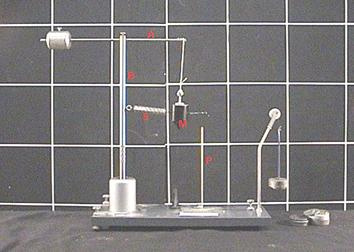### A way to show the magnitude and direction of centripetal forces.

Watch The Video:

Teachable Topics:

• Centripetal Force
• Spring Force
• Force Vectors

Theory:

An object moving in a circle at a constant speed is said to be undergoing "uniform circular motion". Though the speed of the object is not changing, the direction of motion is which implies the object is experiencing acceleration. To cause this acceleration, there must be a force on the object. This force is directed toward the center of the circle, so it is called a "centripetal force".

The machine used in this demonstration (Fig.1) can be used to show some properties of centripetal force. It consists of a mass (M) hanging by a string from a bar on a rotating post (A). The vertical post (B) and the mass are joined by a spring (S), which is responsible for exerting the centripetal force that keeps the mass moving in a circle. Watching the video, one can see not only that the centripetal force is directed toward the center of the circle, but also that it is possible to calculate the magnitude of the centripetal force using this set-up. As the mass rotates faster and faster, it stretches the spring and moves in a circle with a larger and larger radius. Once the circle is large enough, the mass strikes a flexible post (P). Since the spring is exerting the centripetal force, the magnitude of the spring force is equal to the centripetal force. Calculating the spring force at this radius will therefore give the magnitude of the centripetal force.

Apparatus:
• Three metal rods; two that can interlock to form a "T" shape; one rod must hold a mass and the other must have a spring connected to it (A & B
• Various hanging masses
• Mass hanger
• Spring (S)
• Flexible metal rod (P)
• String which connects to mass M and the mass hanger
• Pulley attached to a metal rodFigure 1: Labelled Apparatus

Procedure:

Attach a string to the side of the mass facing the pulley and place it over the pulley.

• Refer to Figure 1 for set-up. Make sure everything is securely attached since the apparatus may end up being spun fairly quickly! (In the filmed demonstration, the entire apparatus is clamped to the table to eliminate wobbling)
• Spin the rotating post faster and faster until the mass, M, just touches the flexible vertical post (P). (This demonstrates the direction of the centripetal motion)

To calculate the magnitude of the centripetal force at the radius of the post:

• Attach a mass hanger to the other end of the string.
• Place hanging masses onto the mass hanger until the spring is stretched enough that the mass, M, touches the vertical post.
• The spring force (and therefore the centripetal force) is then the total mass added to the mass hanger times the acceleration due to gravity, i.e.: Fcentripetal= mg

SAFETY WARNINGS:

• Ensure the mass attached to post A is screwed on tight; otherwise it will fly off when spinning.